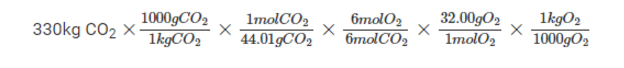# Photosynthesis Formula

Carbohydrates are produced by the process of carbon assimilation which is carried on by the combination of water, carbon dioxide, by the application of external energy, light, and chlorophyll along with the involvement of oxygen.The complete chemical reaction is labeled as photosynthesis.
The general balanced reaction for Photosynthesis Formula according to Kamen and Ruban (1941) is
6CO2        +    12H2O  —–>    C6H12O6   +      6O2   +     6H2O
(Carbon dioxide)    (Water)              (Glucose)        (Oxygen)       (Water)

Photosynthesis is the process of converting energy in which the solar energy is converted into the form of light which is used in the production of carbohydrate molecules.

Here are few solved problems on Photosynthesis.

Problem 1: During Photosynthesis process all green plants synthesis glucose (C6H12O6) and oxygen from carbon dioxide and water. Find out how many kilograms of oxygen is produced from 330kg of carbon dioxide?

Sol:

By substituting the above-mentioned values in the balanced equation of photosynthesis we get

6CO2(g) + 6H2O(l) → C6H12O6(s) + 6O2(g)

In our equation 1 to 1-mole ratio of CO2 to O2Therefore the total amount of produced oxygen from 330kg of carbon dioxide is  240kg.

Problem 2: Write the complete balanced reaction for Photosynthesis both in symbol and word equation.

Sol:

The balanced reaction for photosynthesis in word form is

Carbon dioxide + Water → Glucose  + oxygen.

The balanced reaction for photosynthesis in symbol form is

6CO2 + 6H2O → C6H12O6 + 6O2.

#### 1 Comment

1. Godwill

This information was really helpful to me thanks